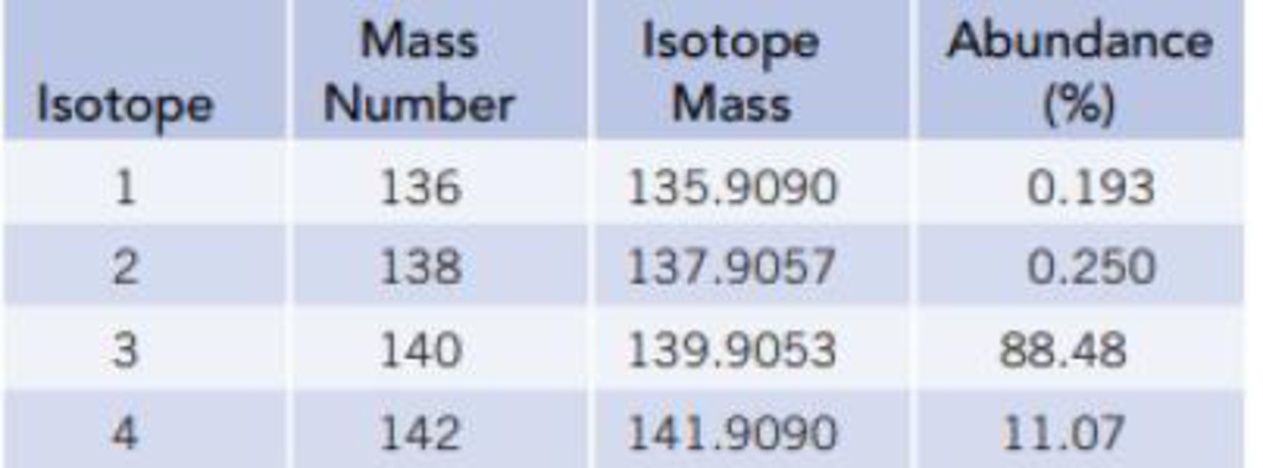Chapter 2, Problem 158GQ

Chapter
Section
Textbook Problem

Mass spectrometric analysis showed that there are four isotopes of an unknown element having the following masses and abundances:Three elements in the periodic table that have atomic weights near these values are lanthanum (La), atomic number 57, atomic weight 138.9055; cerium (Ce), atomic number 58, atomic weight 140.115; and praseodymium (Pr), atomic number 59, atomic weight 140.9076. Using the data above, calculate the atomic weight, and identify the element if possible.

Interpretation Introduction

Interpretation: The average atomic mass and the elements are needed to be determined from the given data’s.

Concept introduction:

Equation for average weight is

average mass=(%abundanceisotop-1100)×(massofisotop-1) +(%abundanceisotop-2100)×(massofisotop-2)+etc

Mass: It is the quantitative measure of a substance. The amount of matter present in substance is expressed as mass. The S.I. unit of mass is kg.

Explanation

There are four isotopes for the element.

The mass of isotope-1 is 135.9090u and its abundance is 0.193%.

The mass of isotope-2 is 137.9057u and its abundance is 0.250%.

The mass of isotope-3 is 139.9053u and its abundance is 88.48%.

The mass of isotope-4 is 141.9090u and its abundance is 11.07%.

Equation for average weight is,

average weight=(%abundanceisotop-1100)×(massofisotop-1) +(%abundanceisotop-2100)×(mass

Still sussing out bartleby?

Check out a sample textbook solution.

See a sample solution

The Solution to Your Study Problems

Bartleby provides explanations to thousands of textbook problems written by our experts, many with advanced degrees!

Get Started

Find more solutions based on key concepts